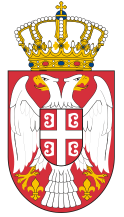Dokument se učitava

# Probability and StatisticsCourse code: 100607 | 6 ECTS credits

Basic information
Level of Studies: Undergraduate applied studies
Year of Study: 2
Semester: 3
Requirements:
Goal: To train students to make decisions in planning or management related to knowledge of probability.
Outcome: At the end of the course, students will be able to design and solve software and process quality problems, analyze data from different sources and understand the importance of empirical methods in software engineering.
Contents of the course
Theoretical instruction:
1. Introduction to Probability and Counting.
2. Conditional Probability and Independence.
3. Discrete Random Variables.
4. Continuous Random Variables.
5. Distributions Basic Terms.
6. Binomial Distribution and Poisson Distribution
7. Normal Distribution.
8. Expectation and Distribution Parameters.
9. Functions of Random Variables.
10. Central Limit Theorem.
11. Random Processes.
12. Introduction to Mathematical Statistics.
13. Parameter Estimation.
14. Testing Statistical Hypothesis.
15. Applied Statistics in Engineering.
Practical instruction (Problem solving sessions/Lab work/Practical training):
1. Practical classes follow the program of lectures and take place through exercises in the computer laboratory, using the software packages Matlab and Maple.
Textbooks and References
1. S.Štrbac-Savić, A. Savić, Verovatnoća i statistika, VIŠER, Beograd, 2013.
2. A. Savić, S. Štrbac-Savić, A. Zeković, Verovatnoća i statistika priručnik za laboratorijske vežbe, VIŠER, Beograd, 2011.
3. S.Cvetković, Elementi teorije verovatnoće i matematičke statistike, Akademska misao Beograd, 2004.
Number of active classes (weekly)
Lectures: 2
Practical classes: 3
Other types of classes: 0
Grading (maximum number of points: 100)
Pre-exam obligations
Points
activities during lectures
0
activities on practial excersises
20
seminary work
0
colloquium
25
Final exam
Points
Written exam
25
Oral exam
30© 2019 School of Electrical and Computer Engineering of Applied Studies, Belgrade
Vojvode Stepe 283, office@viser.edu.rs, +381 11 2471 099